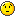# Interpolating TTree 2D data and binning in TH2

Dear Rooters

I have a 2D plot (obtained from TTree) which I’ve dumped into a 2D histogram. I want to interpolate between data points and rebin to access the interpolated data. Using TProfile doesn’t seem to help as it only gives access to the original data, i.e. some bins will have value of 0. I’ve tried using Tgraph, and whilst displaying the interpolated plot is trivial, dumping it into a 2D histogram isn’tSee plots below to illustrate problem. So I guess I have two questions:

(a) is there a way to access intepolated data using TProfile(b) If I went staight from TTree to TGraph, is there a way to dump the interpolated plot into a histogram with an arbitrary number of bins? See script below for one of the many options I tried.Many thanks!

``````ttree ->Draw("y:x");
TGraph *g = new TGraph(nbins,tree->GetV1(),tree->GetV2())
g->Draw("ALP"); // this gives me plot (D)
// to dump into 2D histo
TH2F *h = new TH2F("h","h",nbinsx,x1,x2,nbinsy,y1,y1);
h->Draw();
g->Draw("ALP">>h); // This dosen't work! ``````

See plot:

Well … inventing random syntax rarely works…erm… similar syntax will work for dumping a TTree/Ntuple plot into a histogram, so why not into a Tgraph? (I guess that’s probably a philosophical question - like asking why are apples and pears different)But back to my original question, how can I dump my TGraph plot into a histogram so that I can access the interpolated data. I’ve searched the internet( and previous forum entries)…and the few proposed solutions I found seem really convoluted. Is there an elegant/simple solution that I’m missing. Please I need some help as I’m not expert ROOT.

``````
void FitPeaks()
{

[...]

data->Draw("y:x","","l"); // data is an Ntuple

Int_t  n = data->GetEntries();

Double_t xbins[n];
Int_t i;
for (i=0;i<n;i++) {
data->GetEvent(i);
xbins[i] = x;
}
TH1F *h= new TH1F("h","h",n-1,xbins);

for (i=0;i<n;i++) {
data->GetEvent(i);
h->Fill(x,y);
}

h->SetLineColor(kRed);
h->Draw("hist same");
}
``````

because you do the >> outside of the quotes ! on the option !! …… >> is done on the expression in case of ntuple … totally different ! …

Full code is attached
NSB_detailed.txt (5.44 KB)
FitPeaks.C (572 Bytes)

many thanks. I’ll give it a try!

Tried it. Works fine, but only for TH1 (x axis values increase with increasing n) and not TH2 (because my Y values decrease with increasing n). pity as I need to access Y bin values as well.

All is not lost however… as I’m thinking that a less elegant workaround could be to read in 2 separate Ntuple root files, each generated from the same data sample but with the x and y columns sorted in increasing order respectivelythanks